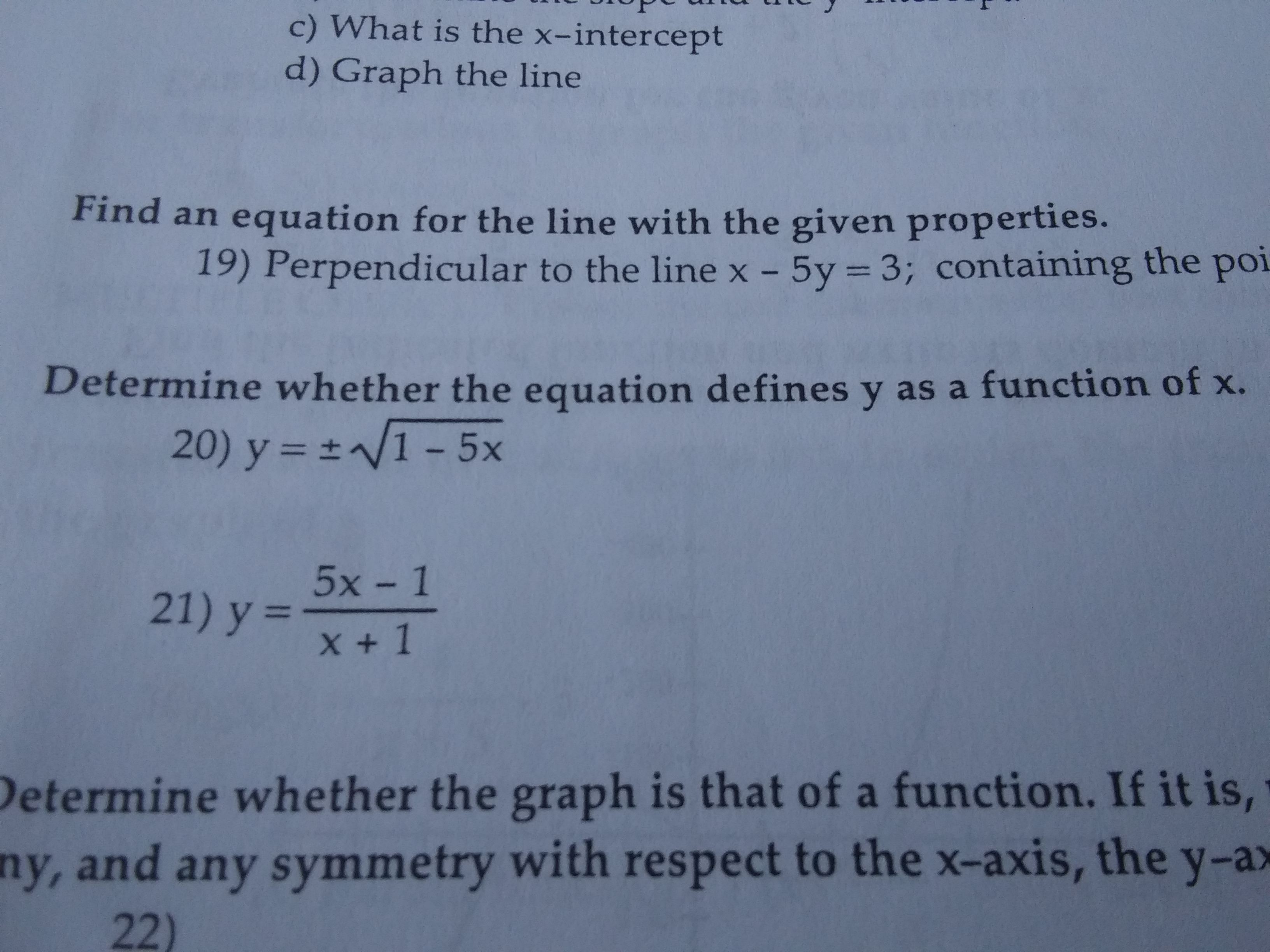# c) What is the x-interceptd) Graph the lineFind an equation for the line with the given properties.19) Perpendicular to the line x - 5y3; containing the poiDetermine whether the equation defines y as a function of x.20) y N1 -5x5x-121) y =X1Determine whe ther the graph is that of a function. If it is,ny, and any symmetry with respect to the x-axis, the y-ax22)

Question

Determine whether the equation defines y as a function of x

1. Y=+/-  square root 1-5x
2. Y= 5x-1/x+1help_outlineImage Transcriptionclosec) What is the x-intercept d) Graph the line Find an equation for the line with the given properties. 19) Perpendicular to the line x - 5y 3; containing the poi Determine whether the equation defines y as a function of x. 20) y N1 -5x 5x-1 21) y = X1 Determine whe ther the graph is that of a function. If it is, ny, and any symmetry with respect to the x-axis, the y-ax 22) fullscreen
check_circle

Since you have submitted two questions, we'll answer the first question. For the second question please submit the question again and specify it.

For one value of x we get two values of y. For example...

### Want to see the full answer?

See Solution

#### Want to see this answer and more?

Solutions are written by subject experts who are available 24/7. Questions are typically answered within 1 hour.*

See Solution
*Response times may vary by subject and question.
Tagged in

### Algebra